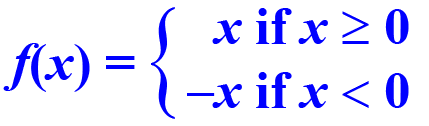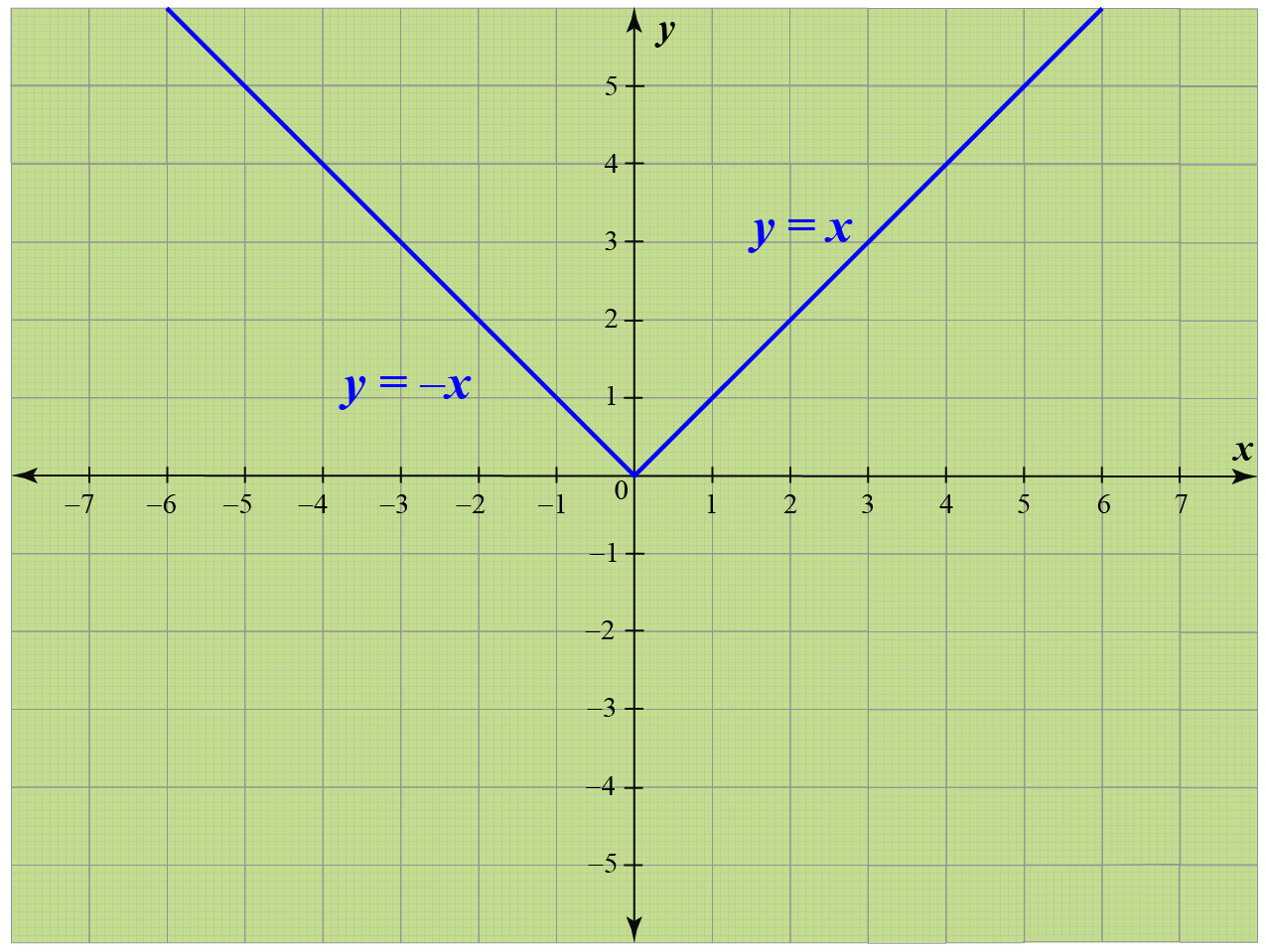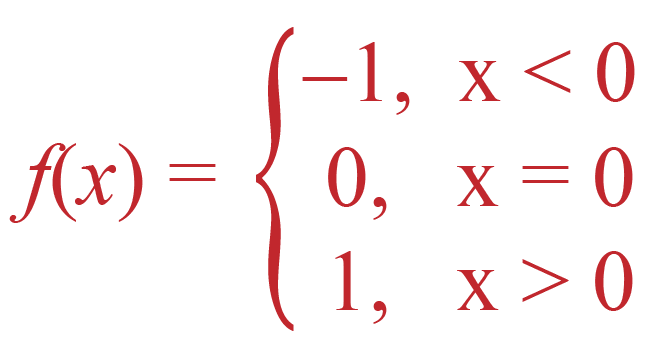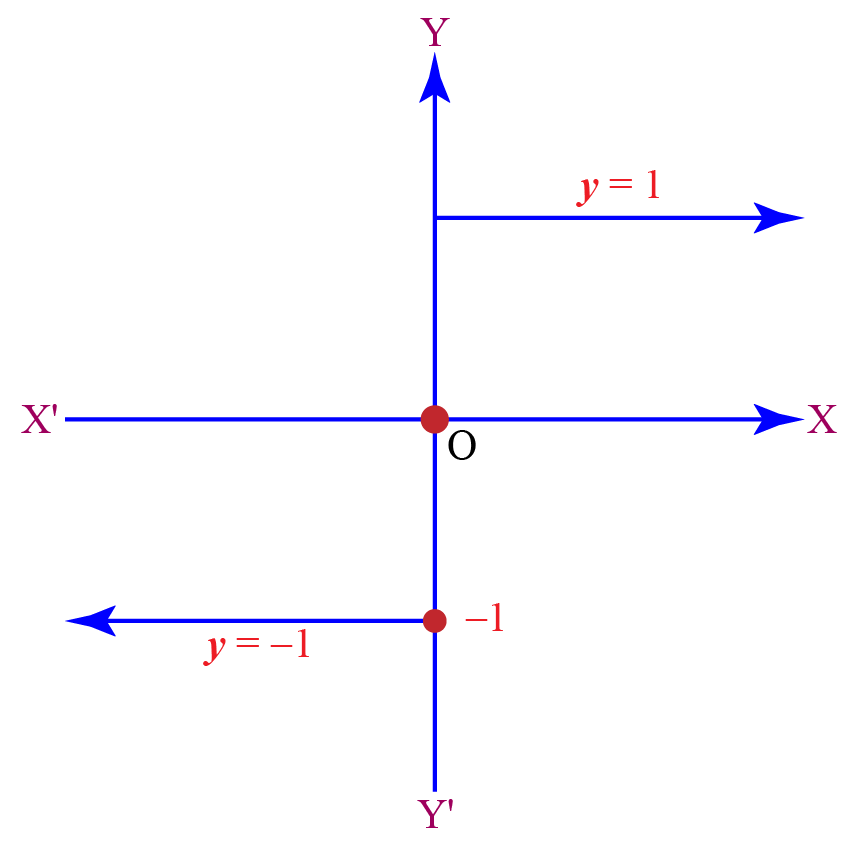# Modulus Function

Modulus Function

A modulus function gives the magnitude of a number irrespective of its sign. It is also called the absolute value function.

In this mini-lesson we will learn about the modulus function definition, calculating modulus for numbers, variables and polynomials along with solved examples and modulus function questions.

Try out the mod function calculator to find the modulus of a number!

## Lesson Plan

 1 What Is Modulus Function? 2 Important Notes on Modulus Function 3 Solved Examples on Modulus Function 4 Tips and Tricks on Modulus Function 5 Interactive Questions on Modulus Function

## What Is Modulus Function?

The modulus of a function, which is also called the absolute value of a function gives the magnitude and absolute value of a number irrespective of the number being positive or negative. It always gives a non-negative value of any number or variable.

It is represented as

\begin{align}y = |x|\end{align}

or

\begin{align}f(x) = |x|\end{align}

where \begin{align}f :R\rightarrow R \end{align} and \begin{align}x \in R\end{align}

\begin{align}|x|\end{align} is the modulus of \begin{align}x\end{align}, where \begin{align}x\end{align} is a non-negative number.

If  \begin{align}x\end{align} is positive then \begin{align}f(x)\end{align} will be of the same value \begin{align}x\end{align}. If \begin{align}x\end{align} is negative, then \begin{align}f(x)\end{align} will be the magnitude of \begin{align}x\end{align}.

To sum up the above lines,This means if the value of \begin{align}x\end{align} is greater than or equal to 0, then the modulus function takes the actual value, but if \begin{align}x\end{align} is less than 0 then the function takes minus of the actual value 'x'.

## How To Calculate The Modulus Function?

The steps to calculate modulus functions are given below.

if \begin{align}x = -3 \end{align}, then

\begin{align}y = f(x) = f(-3) = - (-3) = 3\end{align}, here \begin{align}x\end{align} is less than 0

if \begin{align}x = 4 \end{align}, then

\begin{align}y = f(x) = f(4) = 4\end{align}, here \begin{align}x\end{align} is greater than 0

if \begin{align}x = 0 \end{align}, then

\begin{align}y = f(x) = f(0) = 0\end{align}, here \begin{align}x\end{align} is equal to 0

To sum up, the modulus of a negative number and a positive number is the same number.

## Graph Of Modulus Function

Now let us see how to plot the graph for a modulus function and find its domain and range.

Let us consider x to be a variable, taking values from -5 to 5

 x -5 -4 -3 -2 -1 0 1 2 3 4 5 y = f(x) 5 4 3 2 1 0 1 2 3 4 5

Calculating modulus for the positive values of 'x', the line plotted in the graph is 'y = x'

and for the negative values of 'x', the line plotted in the graph is 'y = -x'.Please note that, we can apply modulus to any real number. The range of the modulus function is the set of non-negative integer variables which is denoted as \begin{align}(0,\infty)\end{align} and the domain of modulus function is R (where R refers to the set of all positive real numbers)

As we discussed modulus is a non-negative value and by this interpretation, we can also say that modulus is the square root of square of a variable.

\begin{align}|x| = \sqrt{x^2}\end{align}

There are a few other non-negative expressions which are enumerated below.

Modulus of an expression or variable = \begin{align}|x|\end{align}

Even root of a expression or variable = \begin{align}x^{\dfrac{1}{2n}}\end{align} where \begin{align}n \in Z\end{align}

Even power of a expression or variable = \begin{align}x^{2n}\end{align} where \begin{align}n \in Z\end{align}

\begin{align}y = 1 - sin\:x; y = 1 - cos\:x \:as \:sin \:x ≤1\: and \:cos\:x ≤1\end{align}

## Signum Function

Signum function is defined as a mathematical function that gives the sign of a real number. The signum function is expressed as follows.The graph of the signum function is as follows.## Modulus of a Complex Number

A complex number is a number which is of the form \begin{align}a + bi \end{align}, where 'a' and 'b' are real numbers and 'i' is an imaginary unit.

Modulus of a complex number \begin{align}z = a + bi \end{align}  can be defined as \begin{align}|z| = \sqrt{a^2 + b^2}\end{align}

## Important Properties of a Modulus Function

### Modulus and Equality

The modulus function always evaluates to a non-negative number for all real values of 'x'. Also equating the modulus function to a negative number is not correct.

\begin{align}|f(x)|=a; \:a>0⇒f(x)=±a \\|f(x)|=a;\:a=0⇒f(x)=0\\|f(x)|=a;a<0 \end{align}

### Modulus and Inequality

Case 1: (If a > 0)

Inequality of a negative number

\begin{align} |f(x)| < a ; a> 0 \Rightarrow -a < f(x) < a \end{align}

Inequality for a positive number

\begin{align} |f(x)| > a ; a> 0 \Rightarrow -a < f(x) > a \end{align}

Case 2: (If a < 0)

\begin{align} |f(x)| < a ; a < 0 \Rightarrow \end{align} - there is no solution for this.

\begin{align} |f(x)| > a ; a < 0 \Rightarrow \end{align} - this is valid for all real values of f(x).

### Property 3:

If x,y are real variables, then

\begin{align} |-x| = |x| \end{align}

\begin{align} |x−y|=0⇔x=y \end{align}

\begin{align}|x+y|≤|x|+|y| \end{align}

\begin{align}|x−y|≥||x|−|y|| \end{align}

\begin{align}|xy|=|x| \times |y| \end{align}

\begin{align}|\dfrac{x}{y}| = \dfrac{|x|}{|y|}; |y| \neq 0\end{align}

Now let us look into some solved modulus function questions to understand better.